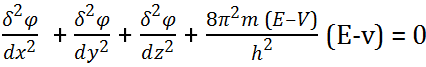Heisenberg’s uncertainty Principle and Quantum Mechanical Model

Heisenberg’s uncertainty principle

According to this principle, “Simultaneous determination of momentum and position of an electron precisely is not possible.”

If uncertainty in measurement of momentum is Δp and uncertainty in measurement of position is Δx, then:
$$\Delta p \times \Delta x \geq \frac{h}{4 \pi}$$
$$or, \Delta (m \times v) \times \Delta x \geq \frac{h}{4 \pi}$$
$$or, m \times \Delta v \times \Delta x \geq \frac{h}{4 \pi}$$
$$\therefore \Delta v \times \Delta x \geq \frac{h}{4 \pi m }$$

Quantum Mechanical Model: Depending upon the De-Broglie’s dual particle of nature and Heisenberg’s uncertainty principle, a new model of atom is proposed called the wave mechanical model.

Wave mechanical model: In this model, the position of the electron in an atom is given by an equation known as Schrodinger’s wave equation.where x, y, z are space coordinates.

E = Total energy of the electron.
v = Potential energy of electron
φ = Wave function which gives the amplitude of the electron wave.

Do you like this article ? If yes then like otherwise dislike :